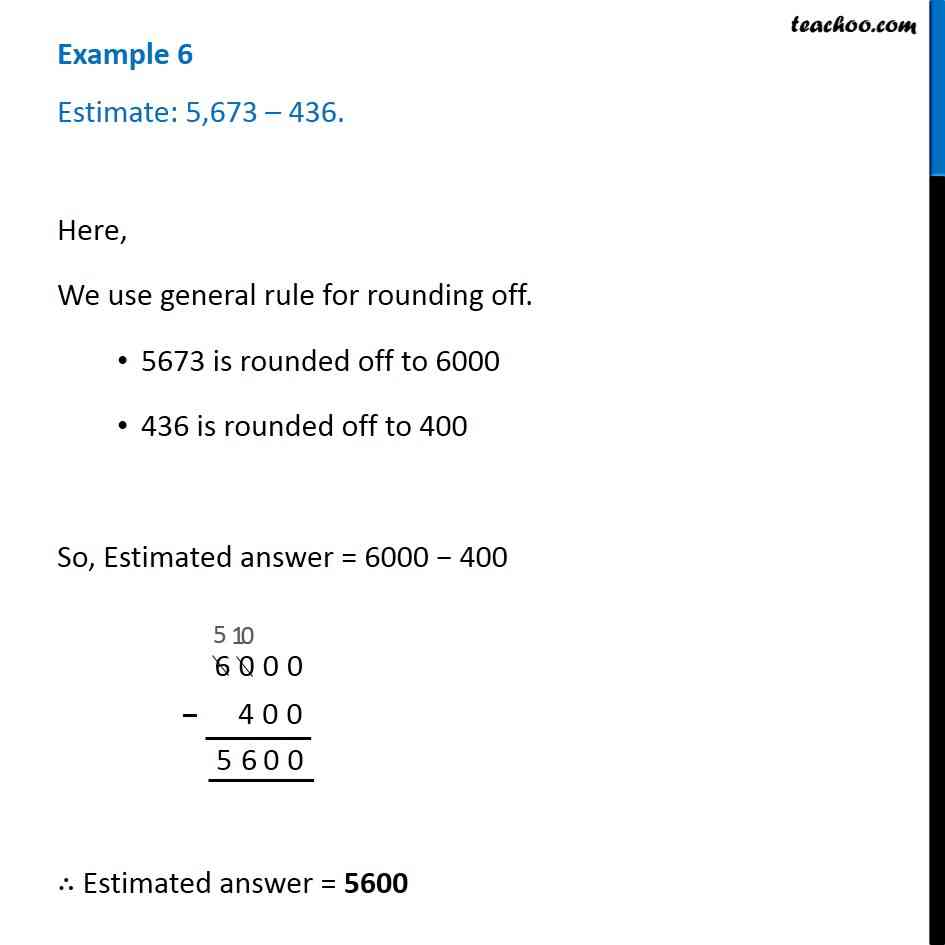1. Chapter 1 Class 6 Knowing our Numbers
2. Concept wise
3. Estimating sum and difference

Transcript

Example 6 Estimate: 5,673 – 436.Here, We use general rule for rounding off. 5673 is rounded off to 6000 436 is rounded off to 400 So, Estimated answer = 6000 − 400 ∴ Estimated answer = 5600